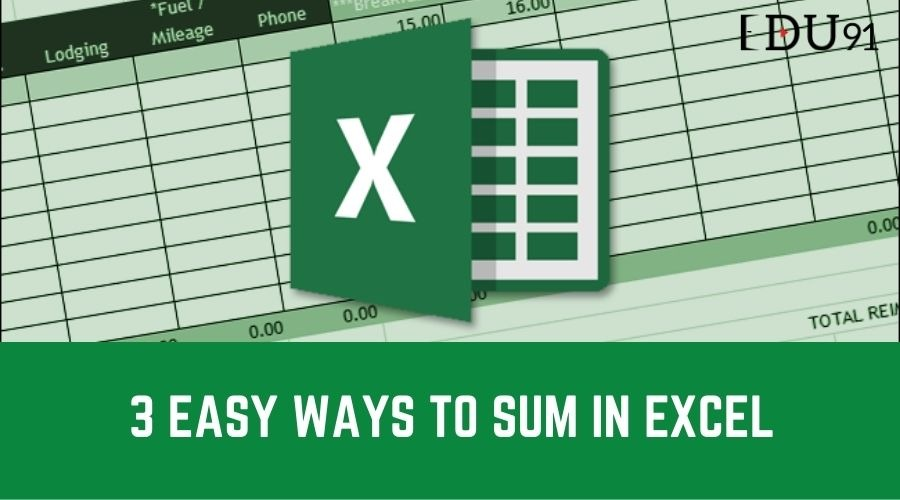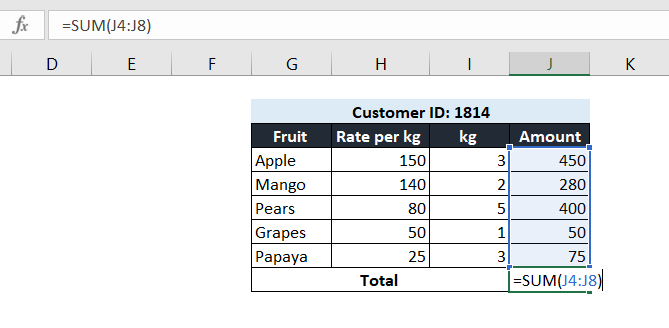excel sum, sum in excel## 3 Easy Ways to Sum in Excel

The SUM function in Excel adds values—numbers, cell references, ranges, arrays, and constants, in any combination. SUM can handle up to 255 individual arguments.

### #1: AutoSum Button

The quickest way to sum a range of cells is to use the AutoSum Button under the Home Tab. It enters a SUM function in the selected cell. The SUM function, in turn, totals one or more numbers in a range of cells.

### #2: Manually typing the SUM function

Instead of using the AutoSum command to insert the SUM function, you can type the function manually.

Syntax: =SUM (number1, [number2], [number3], ...)

The above syntax has one required argument: number 1. As mentioned earlier, the arguments can be cell references or can be typed into the formula.In the above example, there is one argument: a reference to cells J4:J8. Alternatively, you could also give references to each number individually. In that case, there would be 5 arguments: J4, J4, J6, J7 and J8.

Check Out: Excel Club

### #3: Grand Total a range of cells and Keyboard Shortcut

In one quick and easy step, you can calculate the row, column, and grand totals for a range of cells. To do so, select the range of cells, the blank row below the range, and the blank cells in the column to the right. Click the “AutoSum” button on the Home tab. A SUM formula will be automatically entered for each Total.

Alternatively, you can use a keyboard shortcut to calculate the row, column, and grand totals for a range of cells. Select the range of cells, the blank row below the range, and the blank cells in the column to the right, and simply use the shortcut Alt+=. Voila! You have all the rows, columns and grand totals.Shreya Nayak